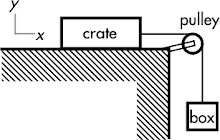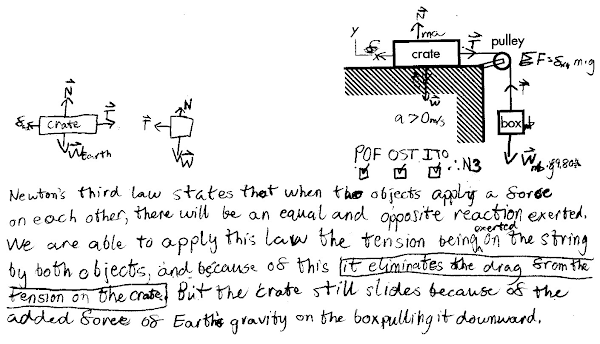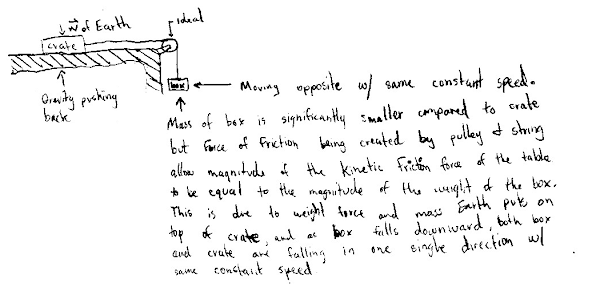## 20141101

### Physics midterm question: crate pulled at constant speed by falling box

Physics 205A Midterm 1, fall semester 2014
Cuesta College, San Luis Obispo, CA

Cf. Giambattista/Richardson/Richardson, Physics, 2/e, Comprehensive Problem 4.118A crate on a horizontal table is attached by a string to a hanging box. The crate is more massive than the box. The string and pulley are ideal, neglect drag, but the table is not frictionless. The crate slides across the table with the same constant speed as the box falling downwards. Discuss why the magnitude of the kinetic friction force of the table on the crate is equal to the magnitude of the weight of the box. Explain your reasoning using free-body diagram(s), the properties of forces, and Newton's laws.

• p:
Correct. Complete free-body diagrams, and discusses/demonstrates:
1. Newton's first law applies to both the constant motion crate and the constant motion box, such that the kinetic friction force on the crate (to the left) is equal in magnitude to the tension force on the crate (to the right); and the tension force on the box (upwards) is equal in magnitude to the weight force on the box (downwards);
2. Newtons' third law applies to the tension force on the crate (to the right) and the tension force on the box (upwards), such they are opposite in direction (relative to the pulley) and equal in magnitude)
3. Thus the kinetic friction force on the crate (to the left) is equal in magnitude to the weight force on the box (downwards).
(Also valid to discuss the crate and box as a combined rigid system due to the properties of an ideal rope and pulley connection, and not need to explicitly discuss Newton's third law as the tension forces would be internal forces within the combined crate/box system.)
• r:
As (p), but argument indirectly, weakly, or only by definition supports the statement to be proven, or has minor inconsistencies or loopholes. Use of either (1) Newton's first law or (2) Newton's third law is only implied or indirectly supported, the other Newton's law discussion is correct/complete.
• t:
Nearly correct, but argument has conceptual errors, or is incomplete. Use of either (1) Newton's first law or (2) Newton's third law is missing, the other Newton's law discussion is correct/complete. Or both Newton's laws discussions are slightly problematic.
• v:
Limited relevant discussion of supporting evidence of at least some merit, but in an inconsistent or unclear manner. Some garbled attempt at applying Newton's laws to a free-body diagram.
• x:
Implementation of ideas, but credit given for effort rather than merit. Approach other than that of applying Newton's laws to a free-body diagram.
• y:
Irrelevant discussion/effectively blank.
• z:
Blank.
Sections 70854, 70855, 73320
Exam code: midterm01m0oU
p: 27 students
r: 14 students
t: 11 students
v: 20 students
x: 2 students
y: 0 students
z: 0 students

A sample "p" response (from student 9178), treating the book and crate as separate objects for the first case:A sample "t" response (from student 4129):A sample "x" response (from student 1991):